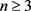# 拟爱因斯坦度量的分类Classification of Quasi-EinsteinMetric

DOI: 10.12677/MP.2019.91002, PDF, HTML, XML, 下载: 560  浏览: 2,133  科研立项经费支持

Abstract: The natural extension of Einstein's metrics for quasi-Einstein metrics (also known as Ricci soliton) has important applications in gauge field theory and super-string theory. There are two important aspects of it: One looking at the influence on the geometry and topology by the quasi-Einstein met-ric structure of the Riemannian manifold; and the other looking at its geometric properties and invariant. In this paper, we are interested in summarizing the classification of it and giving some results about gradient steady and expanding quasi-Einstein metric under the assumption of cur-vature, Weyl tensor and Bach flat.

1. 引言

20世纪80年代，Hamilton  提出了Ricci流的概念，实际上Ricci流最初引进是为了解决3维流形著名的Poincaré猜想(任意单连通的3维闭流形必同胚于3维闭球面)。拟爱因斯坦度量(又称Ricci孤立子)是Ricci流的自相似解  且经常出现在Ricci流方程的奇异点经伸缩变换后的极限中     。一方面，拟爱因斯坦度量的研究有助于更好地理解Ricci流的奇异结构，从而结合几何手术的方法可以得到一些重要的几何和拓扑结果，是研究黎曼流形最有力的工具之一，因此它在几何分析和Ricci流的研究中具有重要的地位。另一方面，从微分方程的角度看拟爱因斯坦度量是爱因斯坦度量的自然推广  ，在相对论、规范场论与超弦理论中有重要的应用，因此其分类对于数学及物理发展均具有重要的研究意义。

1.1. 拟爱因斯坦度量介绍

1.2. 典型的拟爱因斯坦度量

1) $\left({R}^{n},{g}_{0},\frac{|x{|}^{2}}{4}\right)$ 为带有势函数 $f=\frac{|x{|}^{2}}{4}$ 的梯度收缩Ricci孤立子， $Ric+{\nabla }^{2}f=\frac{1}{2}{g}_{0}$

2) $\left({R}^{n},{g}_{0},-\frac{|x{|}^{2}}{4}\right)$ 为势函数是 $f=-\frac{|x{|}^{2}}{4}$ 的梯度扩张Ricci孤立子， $Ric+{\nabla }^{2}f=-\frac{1}{2}{g}_{0}$

2. 梯度稳定拟爱因斯坦度量的分类

2002年，Perelman  提出著名的猜想：非平坦的k-非坍塌梯度稳定拟爱因斯坦度量必为Bryant孤立子。Brendle  最终给出证明：

$n=3$ 时，减弱假设条件下可得一个类似的分类结果：3维完备梯度稳定拟爱因斯坦度量，若满足 $\underset{r\to +\infty }{\mathrm{lim}}\mathrm{inf}\frac{1}{r}{\int }_{{B}_{r}\left(o\right)}R=0$，则 $\left({M}^{3},{g}_{ij}\right)$ 等距于 ${R}^{3}$$R×{\sum }^{2}$ 的一个有限商空间。

Bryant  曾证明存在唯一非平坦的梯度稳定 拟爱因斯坦度量为 ${S}^{n-1}$ 与R的乘积，在此基础上，Catino-Mantegazza  给出以下命题：时，Weyl张量定义为：

${W}_{ijkl}={R}_{ijkl}+\frac{R}{\left(n-1\right)\left(n-2\right)}\left({g}_{ik}{g}_{jl}-{g}_{il}{g}_{jk}\right)-\frac{1}{n-2}\left({R}_{ik}{g}_{jl}-{R}_{il}{g}_{jk}+{R}_{jl}{g}_{ik}-{R}_{jk}{g}_{il}\right)$

Bach张量定义为：

${B}_{ij}=\frac{1}{n-3}{\nabla }^{k}{\nabla }^{l}{W}_{ikjl}+\frac{1}{n-2}{R}_{kl}{W}_{ij}^{kl}$

$n\ge 4$ 时，根据Bach平坦也能得到很多关于稳定拟爱因斯坦度量的分类。特别地，Bach平坦表明局部共形平坦，能否在Weyl张量一阶消失的条件下获得类似的结果依然是一个有待解决的问题。

3维张量 ${D}_{ijk}$ 定义为：

${D}_{ijk}=\frac{1}{n-2}\left({A}_{jk}{\nabla }_{i}f-{A}_{ik}{\nabla }_{j}f\right)+\frac{1}{\left(n-1\right)\left(n-2\right)}\left({g}_{jk}{E}_{il}-{g}_{jk}{E}_{jl}\right){\nabla }_{l}f$

3. 梯度扩张拟爱因斯坦度量的分类

Brendle   证明了具有正截面曲率的梯度稳定拟爱因斯坦度量附加额外条件时必为旋转对称的。在此基础上，扩张情况下Chodosh  证明了：

1) 势函数f为常数函数， ${g}_{ij}$ 为爱因斯坦度量；

2) $\left({M}^{4},{g}_{ij}\right)$ 等距于的 ${R}^{2}×{N}_{\lambda }$ 一个有限商空间，其中 ${N}_{\lambda }$ 为2维黎曼流形且具有常曲率 $\lambda <0$ 、且其势函数为 $f=\frac{\lambda }{2}{|x|}^{2}$

3) $\left({M}^{4},{g}_{ij}\right)$ 为局部共形平坦的。

Ricci流的齐次解相对简单且出现在Ricci流的极限中，长期以来也得到诸多研究及重要进展。此外，在没有爱因斯度量时，齐次Ricci孤立子度量自然是一个非常不错的选择，因此齐次Ricci孤立子空间的分类也一直是微分几何的一个核心问题，其中Jablonski  给出了有关齐次拟爱因斯坦度量的分类定理：任意紧致齐次的拟爱因斯坦度量必为爱因斯坦度量。希望得到的黎曼流形上拟爱因斯坦度量的研究与更为精确的分类能够更好地应用于解决广义相对论及爱因斯坦场方程等问题。

NOTES

*通讯作者。